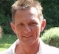## fft/ifft scaling revisitedStarted by 6 years ago6 replieslatest reply 6 years ago1873 views

Hi All,

Judging by Matlab or Octave fft/ifft the scaling applied for (n) resolution is:

fft output is scaled up by (n) for power

ifft is scaled by 1/n for power

Obviously they maintain power unity when crossing both domains.

My question is why they don't keep unity power across one domain e.g. between time domain and frequency domain since we are just moving same signal across.

Regards

[ - ]The requirement is for iDFT(DFT(x)) = x (without rescaling).

Work this through and you will see that the product of the constants before the sums needs to be 1/N. You can decide to put the 1/N before the DFT (which makes sense, since then the DC will be the average of the time domain values), or the 1/N before the IDFT (which, counterintuitively, is what most people do), or 1/sqrt(N) before each.

Y(J)S

[ - ]I'll +1 on some of what's been said before, and just point out that it's often a matter of implementation efficiency.   Scaling by 1/sqrt(N) on both forward and inverse transforms is often done, and may seem more elegant, but requires N more multiplies per transform pair and may require that the arithmetic be floating point.

So often it's much more efficient to put 1/N on either the forward or inverse transform and potentially save a lot of computation or hardware implementation.   As was previously mentioned, putting 1/N on the forward transform yields the input average in the DC bin so there can be some scaling or interpretation advantages in doing it that way.

But it really is up to the implementer as long as you keep track of what's going on.  In some applications you may skip the scaling completely if it is inconsequential to the system.

[ - ]Thanks all for the replies. All make sense. In fact I work with my own scaling well away from unity issues and more to do with signal dynamic range control. But just wondered "in theory" why a signal with power (p) in time domain is converted to frequency domain with (p * n) but obviously it is an implementation issue.

[ - ]I think it is just a question of implementation efficiency.

FFT followed by IFFT will end up in a unit gain transform.

For N=2^n length transform, FFT and IFFT butterfly will just be multiply (exp(+-2i.pi.k/N)) and add/subs, and the last stage of the IFFT will contain a n-bit shift to the right.

[ - ]It is mainly a matter of practical usefulness.
Because, as you propose, it would be possible to normalize the factors to 1.

But, if you do it as it is, this means that you get the same result for the fft, independently of the number of sample rate, which comes very handy.

Now think of streaming: you have a pulse at the beginning, then only zero samples.
The level of the frequency contents decreases with every zero sample, since the pulse's contribution over time has less importance.
After a long time of zeros, if you'd look at the signal on an oscilloscope you'd see almost only nothing. And that's what you get by divising by N.

Therefore I like it as it is :)

[ - ]All the answers have been good.  I just wanted to add the term "unitary matrix" to the discussion for the 1/sqrt(N) scaling.  It is the one that preserves the sum of the squares, which corresponds to preserving "energy".

My preference is for the 1/N factor applied to the forward DFT.  My reasons are as follows:

1) The bin values can be used as the coefficients for sine and cosine functions to make a continous function from the sampled values.

2) The bin values stay roughly the same no matter what the sampling density is for a given interval

3) The concept of the DFT being an average calculation extends beyond the DC bin to all the bins.  This is the topic of my second blog article titled: "DFT Graphical Interpretation: Centroids of Weighted Roots of Unity" which can be found here:

www.dsprelated.com/showarticle/768.php

This was a major "aha moment" on my path to really understanding the DFT.  It remains for me the best explanation of what the DFT really means in terms of a tangible conceptual understanding.  Way more so than understanding it in a Linear Algebra context.

Ced

P.S.  Another common convention I disagree with concerning the DFT is using a lower case 'x' for the signal and an upper case 'X' for the DFT bin values.  I use 'S' and 'Z' respectively in my blog articles.  Also, when a discrete sequence is being discussed, subscript notation should be used, S_n not function notation S(t).  My fiftieth of a dollar's worth.